• 机器人坐标系变换 坐标变换-旋转部分 二维坐标旋转向量和几何表示 对于以前数学学习不好的人来说，在机器人的坐标变换里，总是各种蒙。 这个图片来给你解答。
• 在使用OpenCV3 ...除了采用旋转矩阵描述外，还可以用旋转向量来描述旋转旋转向量的长度（模）表示绕轴逆时针旋转的角度（弧度）。旋转向量旋转矩阵可以通过罗德里格斯（Rodrigues）变换进行转换。 算...
    在使用OpenCV3 stereoRectify()函数处理三维旋转问题时，需要使用旋转向量。通常对于相机的位姿旋转都是采用旋转矩阵的方式来描述的，一个向量乘以旋转矩阵等价于向量以某种方式进行旋转。除了采用旋转矩阵描述外，还可以用旋转向量来描述旋转，旋转向量的长度（模）表示绕轴逆时针旋转的角度（弧度）。旋转向量与旋转矩阵可以通过罗德里格斯（Rodrigues）变换进行转换。
算法过程如下：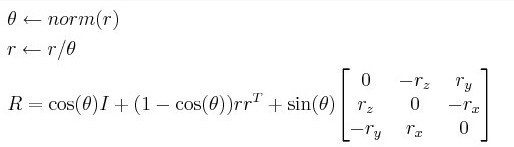式中，norm为求向量的模。反变换也可以很容易的通过如下公式实现：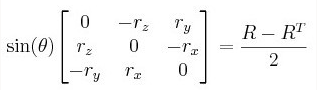OpenCV实现Rodrigues变换的函数为
int cvRodrigues2( const CvMat* src, CvMat* dst, CvMat* jacobian=0 );
src为输入的旋转向量（3x1或者1x3）或者旋转矩阵（3x3）。
dst为输出的旋转矩阵（3x3）或者旋转向量（3x1或者1x3）。
jacobian为可选的输出雅可比矩阵（3x9或者9x3），是输入与输出数组的偏导数。
可以用上述方式法验证以下例子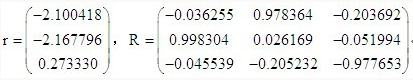验证代码如下：
#include <stdio.h>
#include <cv.h>

void main()
{
int i;
double r_vec={-2.100418,-2.167796,0.273330};
double R_matrix;
CvMat pr_vec;
CvMat pR_matrix;

cvRodrigues2(&pr_vec, &pR_matrix,0);

for(i=0; i<9; i++)
{
printf("%f\n",R_matrix[i]);
}
}

展开全文• 在其他空间中进行右旋90度变换的矩阵表示
在其他空间中进行右旋90度变换的矩阵表示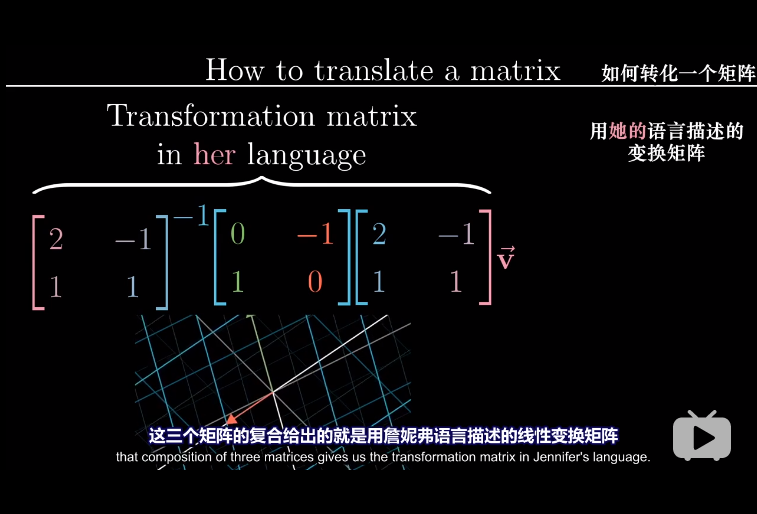展开全文• ## 矩阵、向量的变换

千次阅读 2016-12-29 10:59:57
两个向量的点乘等于它们的数乘结果乘以两个向量之间夹角的余弦值。公式： v¯⋅k¯=||v¯||⋅||k¯||⋅cosθ 如果v¯和k¯都是单位向量，它们的长度会等于1。这样公式会有效简化成： v¯⋅k¯=1⋅1⋅cosθ...
继续  Learn Opengl

向量相乘

点乘

两个向量的点乘等于它们的数乘结果乘以两个向量之间夹角的余弦值。公式：

v¯⋅k¯=||v¯||⋅||k¯||⋅cosθ

如果

v¯
和

k¯
都是单位向量，它们的长度会等于1。这样公式会有效简化成：

v¯⋅k¯=1⋅1⋅cosθ=cosθ

我们使用点乘（dot product）计算方向是否一致。两个单位向量之间的点乘等于这两个向量之间夹角的余弦值。当我们计算出的点乘结果为1，则说明两向量间余弦值为1，即两者夹角为0°，说明两者方向一致，而结果为-1时，说明两者方向相反(180°)。所以我们不必算出最后的角度，仅仅通过点乘结果就可以判断两向量之间方向的关系。
单位向量是指向量长度大小（模）为1的向量，也就是说当向量v满足sqrt(v.x*v.x+v.y*v.y+v.z*v.z)=1时，此向量为单位向量。不要认为单位向量就是(1,1,1)！
如果要使用点乘的结果来表示两向量之间的角度，首先要将两个向量的长度变为单位长度，也就是将两个向量变到单位向量再计算点乘。

点乘的计算是通过将对应分量逐个相乘，然后再把所得积相加来计算的。两个单位向量的（你可以验证它们的长度都为1）点乘会像是这样：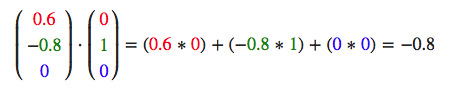要计算两个单位向量间的夹角，我们可以使用反余弦函数

cos−1
，可得结果是143.1度。现在我们很快就计算出了这两个向量的夹角。
叉乘
叉乘只在3D空间中有定义，它需要两个不平行向量作为输入，生成一个正交于两个输入向量的第三个向量。如果输入的两个向量也是正交的，那么叉乘之后将会产生3个互相正交的向量。接下来的教程中这会非常有用。下面的图片展示了3D空间中叉乘的样子：叉乘得到的结果向量使用右手法则得到。运算公式：
设=()，=()。i，j，k分别是X，Y，Z轴方向的单位向量，则：✖️== i·+ j ·+ k·- k·- j·- i·=
(-)
i +
(-)
j
+ (-)
k

矩阵与向量相乘
缩放

对一个向量进行缩放(Scaling)就是对向量的长度进行缩放，而保持它的方向不变。由于我们进行的是2维或3维操作，我们可以分别定义一个

有2或3个缩放变量的向量，每个变量缩放一个轴(x、y或z)。

下面会构造一个变换矩阵来为我们提供缩放功能。我们从单位矩阵了解到，每个对角线元素会分别与向量的对应元素相乘。把向量缩放3倍表示为：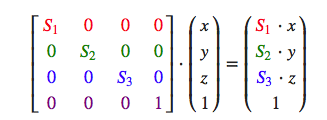注意，第四个缩放向量仍然是1，因为在3D空间中缩放w分量是无意义的。w分量另有其他用途，在后面我们会看到。
位移       位移
(Translation)是在原始向量的基础上加上另一个向量从而获得一个在不同位置的新向量的过程，从而在位移向量基础上
移动
了原始向量。在4×4矩阵上有几个特别的位置用来执行特定的操作，对于位移来说它们是第四列最上面的3个值。如果我们把位移向量表示为

（Tx，Ty, Tz）

，我们就能把位移矩阵定义为：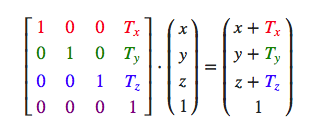所有的位移值都要乘以向量的w行，所以位移值会加到向量的原始值上（想想矩阵乘法法则）。而如果你用3x3矩阵我们的位移值就没地方放也没地方乘了，所以是不行的。

齐次坐标(Homogeneous Coordinates)
向量的w分量也叫齐次坐标。想要从齐次向量得到3D向量，我们可以把x、y和z坐标分别除以w坐标。我们通常不会注意这个问题，因为w分量通常是1.0。使用齐次坐标有几点好处：它允许我们在3D向量上进行位移（如果没有w分量我们是不能位移向量的），而且下一章我们会用w值创建3D视觉效果。
如果一个向量的齐次坐标是0，这个坐标就是方向向量(Direction Vector)，因为w坐标是0，这个向量就不能位移（译注：这也就是我们说的不能位移一个方向）。

旋转

下图中展示的2D向量是k向右旋转72°得到的：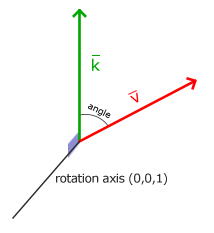旋转矩阵在3D空间中每个单位轴都有不同定义，旋转角度用θ表示：
沿x轴旋转：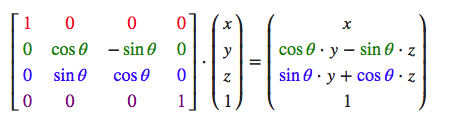沿y轴旋转：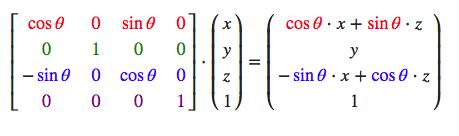沿z轴旋转：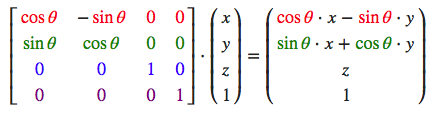利用旋转矩阵我们可以把我们的位置向量沿一个单位轴进行旋转。也可以把多个矩阵结合起来，比如先沿着x轴旋转再沿着y轴旋转。但是这会很快导致一个问题——万向节死锁（Gimbal Lock）。我们不会讨论它的细节，但是一个更好的解决方案是沿着任意轴比如(0.662, 0.2, 0.7222)（注意这是个单位向量）旋转，而不是使用一系列旋转矩阵的组合。这样一个旋转矩阵是存在的，（Rx，Ry，Rz）代表任意旋转轴：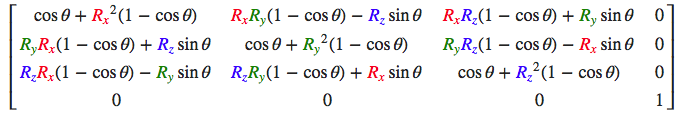避免万向节死锁的真正解决方案是使用四元数(Quaternion)，它不仅安全，而且计算更加友好。


展开全文矩阵 transform
• 用 opencv 进行过双目相机标定的同学都知道，单目标定 calibrateCamera() 函数能够对每一张标定图像计算出一对 rvec 和 tvec，即旋转平...同样是坐标变换，平移倒总是向量，但旋转怎么有时是向量，有时又是矩阵呢？...
用 opencv 进行过双目相机标定的同学都知道，单目标定 calibrateCamera() 函数能够对每一张标定图像计算出一对 rvec 和 tvec，即旋转平移向量，代表世界坐标系到相机坐标系的转换关系。而 stereoCalibrate() 函数则可以计算出旋转矩阵 R 和平移向量 T，代表左右相机坐标系之间的转换关系。同样是坐标变换，平移倒总是向量，但旋转怎么有时是向量，有时又是矩阵呢？

旋转矩阵
参考csxiaoshui的博客，三维旋转变换可以看成是矩阵乘法运算，即：

⎡⎣⎢x′y′z′⎤⎦⎥=R∗⎡⎣⎢xyz⎤⎦⎥

[

x

′

y

′

z

′

]

=

R

∗

[

x

y

z

]

\begin{bmatrix}x' \\ y' \\ z'\end{bmatrix} = R * \begin{bmatrix}x \\ y \\ z\end{bmatrix}
其中

R

R

$R$ 就是三阶的旋转矩阵。仅仅考虑绕 X$X$$X$、

Y

Y

$Y$ 或 Z$Z$$Z$ 单个轴旋转

θ

θ

$\theta$ （右手螺旋），

R

R

$R$ 分别为：
RX=⎡⎣⎢1000cosθsinθ0−sinθcosθ⎤⎦⎥RY=⎡⎣⎢cosθ0−sinθ010sinθ0cosθ⎤⎦⎥RZ=⎡⎣⎢cosθsinθ0−sinθcosθ0001⎤⎦⎥${R}_{X}=\left[\begin{array}{ccc}1& 0& 0\\ 0& cos\theta & -sin\theta \\ 0& sin\theta & cos\theta \end{array}\right]\phantom{\rule{0ex}{0ex}}{R}_{Y}=\left[\begin{array}{ccc}cos\theta & 0& sin\theta \\ 0& 1& 0\\ -sin\theta & 0& cos\theta \end{array}\right]\phantom{\rule{0ex}{0ex}}{R}_{Z}=\left[\begin{array}{ccc}cos\theta & -sin\theta & 0\\ sin\theta & cos\theta & 0\\ 0& 0& 1\end{array}\right]$
R_X=\begin{bmatrix} 1&0&0\\ 0&cos\theta&-sin\theta\\ 0&sin\theta&cos\theta \end{bmatrix}\\
R_Y=\begin{bmatrix} cos\theta&0&sin\theta\\ 0&1&0\\ -sin\theta&0&cos\theta \end{bmatrix}\\
R_Z=\begin{bmatrix} cos\theta&-sin\theta&0\\ sin\theta&cos\theta&0\\ 0&0&1 \end{bmatrix}
绕任意轴旋转则可以分解成绕三个坐标轴旋转的叠加，最终得到的旋转矩阵

R

R

$R$ 便是上述三个矩阵的乘积。

矩阵运算显然是计算机三维坐标变换最简单方便的计算方法，因此在 opencv、opengl、工业机器人等开发中，提到位姿旋转变换，多半用的是旋转矩阵。然而，用矩阵来表示一个旋转关系有两个缺点：
首先，通过旋转矩阵不能直观地看出旋转的方向和角度，假设给定一个旋转矩阵，要求旋转方向不变，旋转角度变成一半，那么新的旋转矩阵计算起来就比较麻烦了。
另一方面，旋转变换本身只有3个自由度，但旋转矩阵有9个元素，因此旋转矩阵中的元素不是相互独立的，这在非线性优化中会带来问题。

旋转向量

向量旋转公式最早由 Rodrigues 提出，用一个三维向量来表示三维旋转变换，该向量的方向是旋转轴，其模则是旋转角度。百度百科中有其详细的介绍与推导，我在这边只列一下最重要的公式。
设旋转向量的单位向量为 r$r$$r$，模为

θ

θ

$\theta$。三维点（或者说三维向量）

p

p

$p$ 在旋转向量 r$r$$r$ 的作用下变换至

p′

p

′

$p'$，则：

p′=cosθ⋅p+(1−cosθ)(p⋅r)r+sinθ⋅r×p

p

′

=

cos

⁡

θ

⋅

p

+

(

1

−

cos

⁡

θ

)

(

p

⋅

r

)

r

+

sin

⁡

θ

⋅

r

×

p

p'=\cos\theta\cdot p+(1-\cos\theta)(p\cdot r)r+\sin\theta\cdot r \times p
显然，旋转向量代表的变换关系十分直观，但运算上要比矩阵形式更加复杂。

相互转换
用矩阵形式和向量形式表示旋转变换各有优劣势，因此经常需要来回转换，这里跟数学中的李群与李代数有所关联，本人目前还一知半解，可以参考半闲居士的博客。  opencv 中有函数 Rodrigues() 用于旋转矩阵和旋转向量的转换。参照opencv文档，设旋转向量的单位向量

r=[rx ry rz]T

r

=

[

r

x

r

y

r

z

]

T

$r=[r_x\ r_y\ r_z]^T$，旋转角度为

θ

θ

$\theta$，对应的旋转矩阵为

R

R

$R$，则 r$r$$r$ 到

R

R

$R$ 的转换是：
R=cosθI+(1−cosθ)rrT+sinθ⎡⎣⎢0rz−ry−rz0rxry−rx0⎤⎦⎥$R=\mathrm{cos}\theta I+\left(1-\mathrm{cos}\theta \right)r{r}^{T}+\mathrm{sin}\theta \left[\begin{array}{ccc}0& -{r}_{z}& {r}_{y}\\ {r}_{z}& 0& -{r}_{x}\\ -{r}_{y}& {r}_{x}& 0\end{array}\right]$
R = \cos\theta I+(1- \cos\theta) r r^T+\sin\theta
\begin{bmatrix}
0&-r_z&r_y\\
r_z&0&-r_x\\
-r_y&r_x&0
\end{bmatrix}
其中

I

I

$I$ 是三阶单位矩阵。反过来 R$R$$R$ 到

r

r

$r$ 的转换则可以利用等式：
R−RT2=sinθ⎡⎣⎢0rz−ry−rz0rxry−rx0⎤⎦⎥$\frac{R-{R}^{T}}{2}=\mathrm{sin}\theta \left[\begin{array}{ccc}0& -{r}_{z}& {r}_{y}\\ {r}_{z}& 0& -{r}_{x}\\ -{r}_{y}& {r}_{x}& 0\end{array}\right]$
\frac{R-R^T}{2}=\sin\theta
\begin{bmatrix}
0&-r_z&r_y\\
r_z&0&-r_x\\
-r_y&r_x&0
\end{bmatrix}
opencv 官方文档中的原话是：

A rotation vector is a convenient and most compact representation of a rotation matrix (since any rotation matrix has just 3 degrees of freedom). The representation is used in the global 3D geometry optimization procedures like calibrateCamera, stereoCalibrate, or solvePnP .

称旋转向量是旋转矩阵方便而且最紧凑的表示方法，被用于一些需要全局三维几何优化的函数中。
展开全文Rodrigues
• 旋转矩阵用一个矩阵来表示空间中的旋转变换关系。 四元数用４个变量来表示旋转（增加一个纬度），可以避免万向节锁现象。 一、Eigen中旋转对象之间的转换 （1）旋转向量->旋转矩阵 （2）旋转向量->四元...
• 三维空间刚体运动的描述方法有：旋转矩阵、变换矩阵、旋转向量、欧拉角和四元数，接下来将逐一介绍它们 一、旋转矩阵 点、向量、坐标系 * 点——存在于三维空间之中，点和点组成向量，点本身由原点指向它的...四元数 欧拉角
• 二维旋转变换 下面是一个简单的绕原点旋转变换的图。 关于图形变换我们关注的都是怎么得到变换之后的坐标，而对于变换后的坐标，很多教材上都只有一个简略的结果，并不会给出详细的推导过程。今天学习旋转变换的...图形变换 计算机图形学
• ## 二维向量旋转

千次阅读 2017-10-18 15:28:31
在二维坐标系中,一个位置向量旋转公式可以由三角函数的几何意义推出. 比如上图所示是位置向量R逆时针旋转角度B前后的情况.  在左图中,我们有关系： 　x0 = |R| * cosA => cosA = x0 / |R| 　y0 = |R| *...
• 除了采用旋转矩阵描述外，还可以用旋转向量来描述旋转旋转向量的长度（模）表示绕轴逆时针旋转的角度（弧度）。旋转向量旋转矩阵可以通过罗德里格斯（Rodrigues）变换进行转换。 OpenCV实现Rodrigues变换的函数...opencv
• 处理三维旋转问题时，通常采用旋转矩阵的方式来描述旋转变换。旋转矩阵有以下两种方式得到。 物体在三维空间中的旋转，可以被分为解为在直接坐标系下，分别先后围绕x,y,z坐标轴旋转得到。旋转的角度也就是我们常...
• 根据问题：向量(a,b)在平面上绕原点逆时针旋转角度α后的坐标为? A(a,b),a=rcost,b=rsint A按逆时针方向旋转α,得到B(X,Y) 有：X=rcos(t+α)=r*costcosα-r*sintsinα=acosα-bsinα　Y=rsin(t+α)=r*costsinα+r*...
• 任意旋转可用一个旋转轴和一个旋转角度来表示旋转向量旋转向量的方向与旋转轴一致...则旋转向量可用k*n表示。使用库eigen可用下面来表示 Eigen::AngleAxisd rotationVector(30,Eigen::Vector3d(0,1,0)); 即绕y
• ## 旋转变换矩阵推导

千次阅读 2020-06-06 17:20:46
先介绍二维旋转变换，然后由此推广到三维重绕坐标轴旋转，再推广得到绕三维任意轴旋转的罗德里格斯旋转公式。 1.二维旋转变换   如果要求PPP逆时针旋转θ\thetaθ得到的P′P^{'}P′，记QQQ为PPP逆时针旋转90°...
• 1、在二维坐标系中，一个向量可以使用三角函数...2、右图是将左图中向量逆时针旋转B之后得到的向量，它的向量表示为： x1 = |R| * cos(A + B) = |R| * cosA * cosB - |R|* sinA * sinB y1 = |R| * sin(A + B) ...
• ## 四元数与旋转变换

千次阅读 2017-06-30 17:03:33
问题还是来源于课本内容，在图形学课本中讲到三维变换矩阵的时候引入了四元数，但是同样没有说明四元素是如何旋转三维空间里向量的原因。通过查找维基百科，这个问题完全可以。 首先简单介绍四元数的表示形式： ...四元数
• ## 旋转变换（一）旋转矩阵

万次阅读 多人点赞 2017-03-27 17:15:37
本文主要介绍了计算机图形学中的旋转的概念和矩阵的描述方式，包括二维和三维旋转矩阵的推导过程图形
• 在二维坐标系中,一个位置向量旋转公式可以由三角函数的几何意义推出.比如上图所示是位置向量R逆时针旋转角度B前后的情况. 在左图中,我们有关系：　x0 = |R| * cosA => cosA = x0 / |R|　y0 = |R| * sinA => ...
• ## C++实现向量的旋转

千次阅读 2017-11-01 15:29:53
博记：通过输入旋转角度得到。...，经过旋转之后得到的向量matrix_result[][]表示； 程序实现如下： #include "iostream" #include #include #include #include #include #include #include #include usc++ matrix
• 坐标变换是实际导航与定位中必不可少的一环，而其中旋转变换至关重要，本文对学习过程中关于坐标变换内容做一点总结，以下是本文提供的转换关系。 说明：旋转矩阵和四元数提供与其他三种旋转表示的转换关系，欧拉角...计算机视觉 定位
• 已知直角坐标系中的两个单位向量，现在想知道其中一个向量a，变换到另一个向量b，所...1、假设a和b都是单位向量，a叉乘b得到旋转轴，a点乘b再求arccos得到旋转角度，从而得到四元数表示 2、将四元数转换为欧拉角，...四元数 欧拉角
• 旋转矩阵用一个矩阵来表示空间中的旋转变换关系。 四元数用４个变量来表示旋转（增加一个纬度），可以避免万向节锁现象。具体转换公式可参考《视觉slam十四讲》这本书。下面的程序为使用Eigen库进行转换。 （1）...
• 深入浅出讲解旋转变换矩阵 近来本人在自学《机器人学基础》一书，起初自以为理解了旋转变换本质，后来发觉最初的理解是不准确或者说完全错误的。于是上网查找了许多关于旋转矩阵以及方向余弦的相关文章，但发现他们......